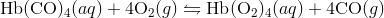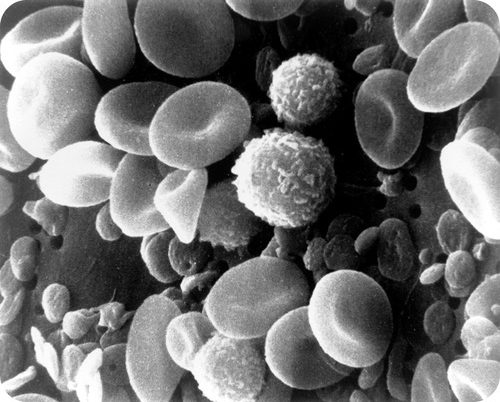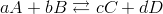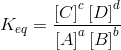## Equilibrium Constant

### Learning Objectives

• Define equilibrium constant.
• Write the equation for the general equilibrium constant.

### What is carbon monoxide poisoning?

Red blood cells transport oxygen to the tissues so they can function. In the absence of oxygen, cells cannot carry out their biochemical responsibilities. Oxygen moves to the cells attached to hemoglobin, a protein found in the red cells. In cases of carbon monoxide poisoning, CO binds much more strongly to the hemoglobin, blocking oxygen attachment and lowering the amount of oxygen reaching the cells. Treatment involves the patient breathing pure oxygen to displace the carbon monoxide. The equilibrium reaction shown below illustrates the shift toward the right when excess oxygen is added to the system:### Equilibrium Constant

Consider the hypothetical reversible reaction in which reactants A and B react to form products C and D. This equilibrium can be shown below, where the lower case letters represent the coefficients of each substance.As we have established, the rates of the forward and reverse reactions are the same at equilibrium, and so the concentrations of all of the substances are constant. Since that is the case, it stands to reason that a ratio of the concentrations for any given reaction at equilibrium maintains a constant value. The equilibrium constant (Keq) is the ratio of the mathematical product of the concentrations of the products of a reaction to the mathematical product of the concentrations of the reactants of the reaction. Each concentration is raised to the power of its coefficient in the balanced chemical equation. For the general reaction above, the equilibrium constant expression is written as follows:The concentrations of each substance, indicated by the square brackets around the formula, are measured in molarity units (mol/L).

The value of the equilibrium constant for any reaction is only determined by experiment. As detailed in the above section, the position of equilibrium for a given reaction does not depend on the starting concentrations and so the value of the equilibrium constant is truly constant. It does, however, depend on the temperature of the reaction. This is because equilibrium is defined as a condition resulting from the rates of forward and reverse reactions being equal. If the temperature changes, the corresponding change in those reaction rates will alter the equilibrium constant. For any reaction in which a Keq  is given, the temperature should be specified.

### Summary

• The equilibrium constant for a reversible reaction is described.

### Practice

1. What is a homogeneous equilibrium?
2. What is a heterogeneous equilibrium?
3. What goes in the numerator in an equilibrium expression?
4. Why is it important to write your equilibrium equation out before setting up the equilibrium constant?

### Review

1. What does the equilibrium constant tell us?
2. What does it mean if the Keq is > 1?
3. What does it mean if the Keq is < 1?
4. Does the position of equilibrium depend on the starting concentrations?

## Glossary

• equilibrium constant (Keq) : The ratio of the mathematical product of the concentrations of the products of a reaction to the mathematical product of the concentrations of the reactants of the reaction. Each concentration is raised to the power of its coefficient in the balanced chemical equation.